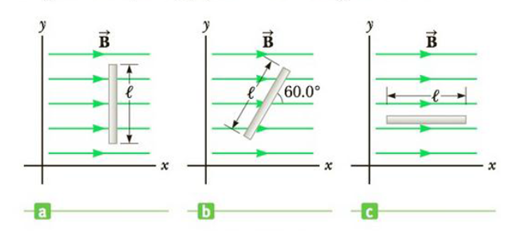Chapter 20, Problem 3P

Chapter
Section
Textbook Problem

Figure P20.3 shows three edge views of a square loop with sides of length ℓ = 0.250 m in a magnetic field of magnitude 2.00 T. Calculate the magnetic flux through the loop oriented (a) perpendicular to the magnetic field, (b) 60.0° from the magnetic field, and (c) parallel to the magnetic field.Figure P20.3

(a)

To determine
The flux when the loop is perpendicular to the magnetic field.

Explanation

Given info: The magnetic field of (B) is 2.00 T. Length of the square loop (l) is 0.250 m.

Formula to calculate flux is,

ϕB=BAcosθ

• θ is the angle between the field and area vector.

The area of the square loop is,

A=l2

Therefore,

ϕB=Bl2cosθ

Substitute 2.00 T for B,0.250 m for l and 0ο for θ

(b)

To determine
The flux when the loop makes 60ο angle with the magnetic field.

(c)

To determine
The flux when the loop is parallel to the magnetic field.

Still sussing out bartleby?

Check out a sample textbook solution.

See a sample solution

The Solution to Your Study Problems

Bartleby provides explanations to thousands of textbook problems written by our experts, many with advanced degrees!

Get Started

Find more solutions based on key concepts